• ## simulink实现ESO（扩张状态观测器）

万次阅读 多人点赞 2018-11-16 16:40:15
1、ADRC的控制框图如下：主要包括跟踪微分器（TD）、非线性状态误差反馈（NLSEF）、扩张状态观测器ESO）。 2、ESO的公式： 3、用simulink搭建eso，如图所示： 4、将上述eso封装为subsystem，输入输出如下...
ADRC最核心的创新点在于ESO，与PID相比，不仅基于误差控制，还基于扰动进行控制量计算。

1、ADRC的控制框图如下：主要包括跟踪微分器（TD）、非线性状态误差反馈（NLSEF）、扩张状态观测器（ESO）。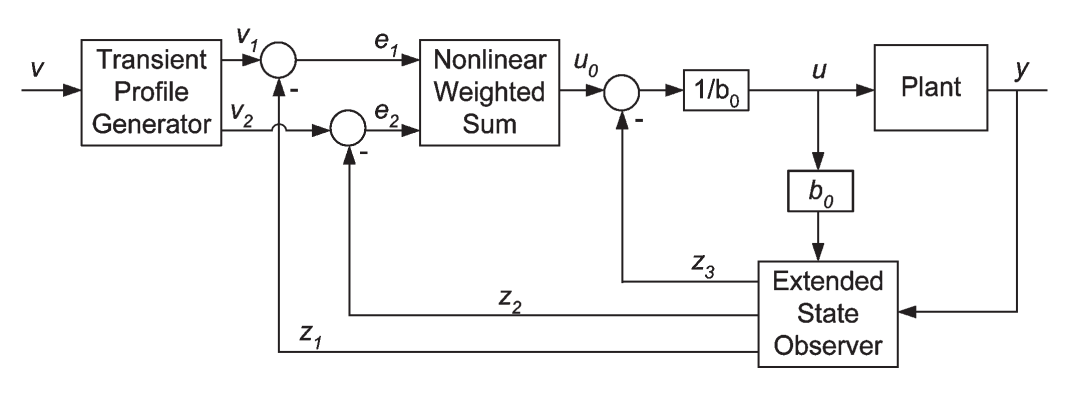2、ESO的公式：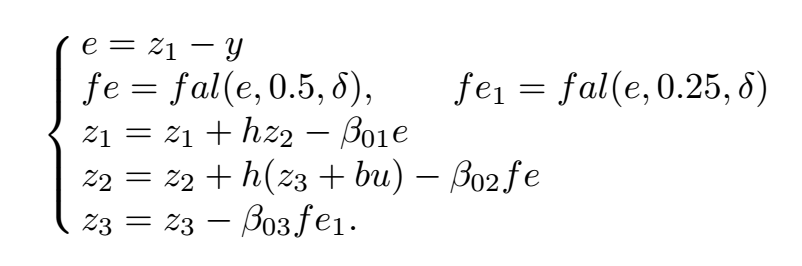3、用simulink搭建eso，如图所示：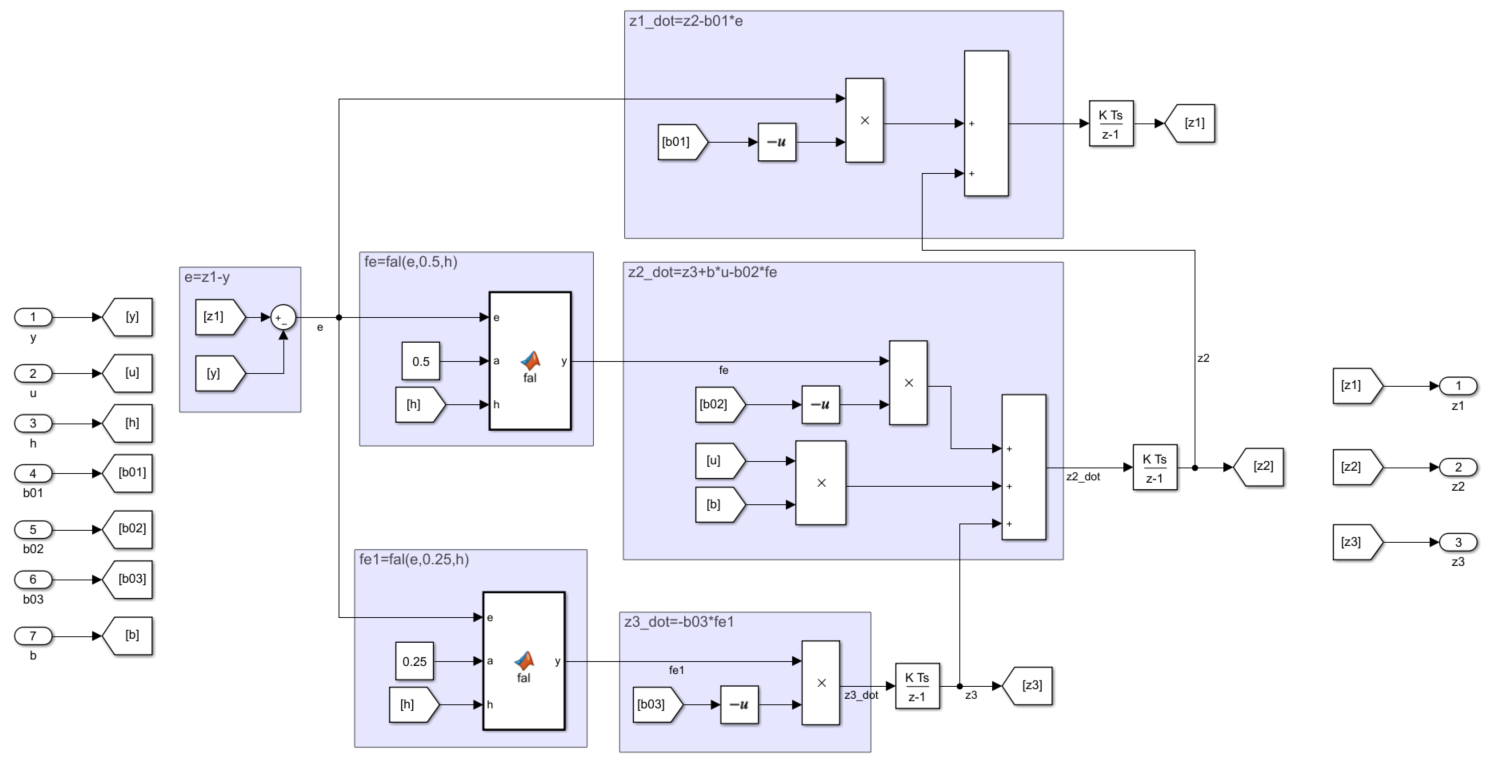4、将上述eso封装为subsystem，输入输出如下所示：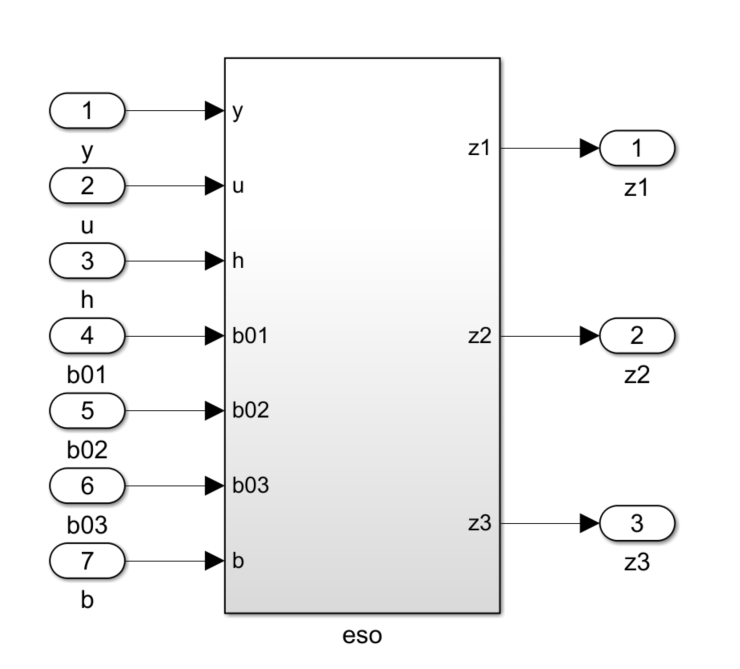展开全文• Simulink实现ESO扩张状态观测器） ADRC最核心的创新点在于ESO，与PID相比，不仅基于误差控制，还基于扰动进行控制量计算。 1、ADRC的控制框图如下：主要包括跟踪微分器（TD）、非线性状态误差反馈（NLSEF）、...
Simulink实现ESO（扩张状态观测器）

ADRC最核心的创新点在于ESO，与PID相比，不仅基于误差控制，还基于扰动进行控制量计算。

1、ADRC的控制框图如下：主要包括跟踪微分器（TD）、非线性状态误差反馈（NLSEF）、扩张状态观测器（ESO）。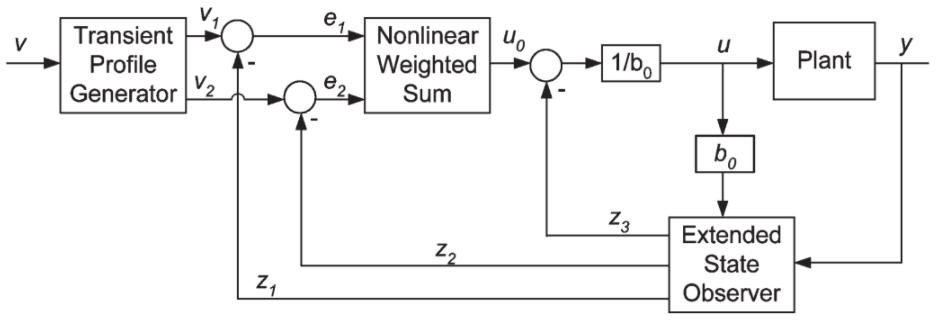2、ESO的公式：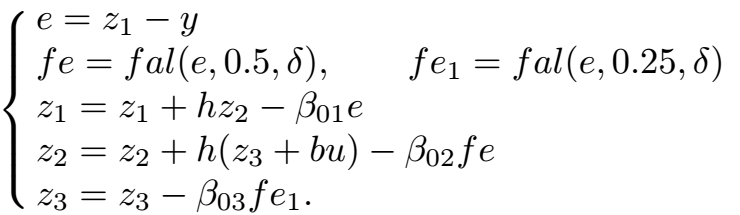3、用simulink搭建eso，如图所示：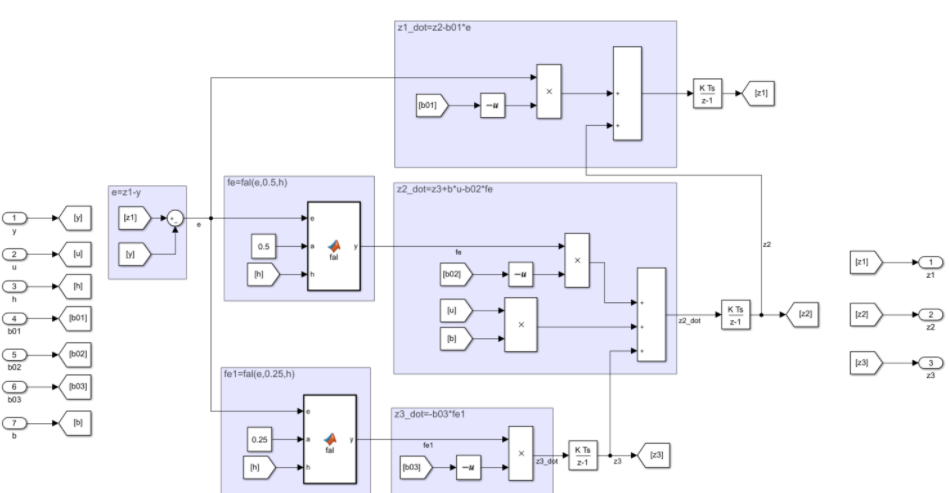4、将上述eso封装为subsystem，输入输出如下所示：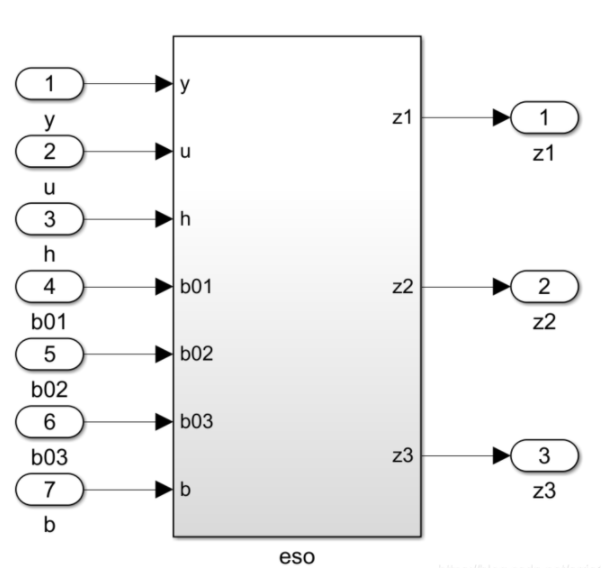展开全文Simulink
• 研究自抗扰控制方法中的扩张状态观测器(ESO). 通过频域分析可得出该观测器性能随频率升高而逐渐衰 减的结论, 衰减程度取决于观测器的参数和系统的采样频率, 并指出对现有的参数配置方法加以改进可以获得补偿 ...
• 首先通过将系统的不确定项以及外部扰动作为扩张状态,设计线性扩张状态观测器(ESO)对系统的总扰动进行估计;然后,在控制器中引入对扩张状态的估计值,对系统的总扰动进行补偿,设计了动态抗饱和补偿器,将控制器、观测器...
• 扩张状态观测器(ESO) 作为自抗扰控制(ADRC) 的核心组件, 其自身及高阶扩展形式的性能分析与评估至关重要. 借助Lyapunov 逆定理证明了任意扩张阶数下线性扩张状态观测器(LESO) 重构状态误差的收敛性, 并得出了观测...
• 在测量噪声条件下,研究基于扩张状态观测器(ESO)的参数辨识方法和实际应用.结合仿真与实验,分析测量噪声对ESO观测状态输出的影响.为了提高实际应用中的辨识效果,通过引入积分型滤波器对观测状态中的噪声进行抑制.所...
• 针对ESO参数不易整定的问题，研究了扩张状态观测器(ESO)参数优化的李雅普诺夫函数法．首先建立关于误差的线性定常状态方程和误差指标函数J，在误差指标函数J，中综合考虑了跟踪误差的权重和系统的稳定性以及实际运行...
• 在建立了精确飞轮开环系统模型的基础上，设计了二阶非线性连续扩张状态观测器ESO(Extended State Observer).将飞轮故障视为系统外扰，并假设其余外扰是小量可忽略，则利用此ESO不仅能实时得到飞轮开环系统的状态量，...
• 基于面贴式永磁同步电机(SPMSM) 的数学模型,...了扩张状态观测器(ESO) 以实时估计控制系统的外界负载扰动, 及时调整控制量, 有效减小滑模变结构中的趋近率 参数. 理论分析及仿真结果验证了该控制方法的有效性.</p>
• 扩张状态观测器(extended state observer, ESO)是自抗扰控制(active disturbance rejection control, ADRC)的核心组成部分，在自抗扰控制的相关文献中大都会反复出现ESO和ADRC这两个英文缩写词。关于ADRC的具体思想...
扩张状态观测器(extended state observer, ESO)是自抗扰控制(active disturbance rejection control, ADRC)的核心组成部分，在自抗扰控制的相关文献中大都会反复出现ESO和ADRC这两个英文缩写词。关于ADRC的具体思想和设计方法可以参见其发明者韩京清的专著，不过，专著中更多地从工程角度对ADRC进行分析，而本文则主要是分析ESO论文证明的套路，也作为自己学习ADRC的一个总结，涉及到的文献则主要为和。
ESO的主要思想在于将包括干扰在内的系统中所有不确定的部分统一视为系统的一个新状态，即对原系统扩张了一个状态，然后设计观测器去估计这个状态。一旦实现对这个新状态的估计，那么在后面的控制律中直接将这个估计值减去，就认为达到了消除不确定性的目的，然后就可以采用任何能够保证剩下已知部分模型稳定的控制律来保证控制性能。从这个角度说，ESO其实承担了ADRC的绝大部分压力，ADRC有效的前提是ESO能够实现对扩张状态快速有效的估计。需要指出的是，ESO在设计过程中也借鉴了现有观测器的经验，而且与HK Khalil的专著中提到的高增益观测器有类似之处，本质上均属于奇异摄动，即需要观测器的状态足够快。特别地，在线性情形下，ESO与文献中的扩张高增益观测器具有相同形式，可见奇妙的思想总是相通的。
下面以文献为主要内容，对ESO的收敛性分析进行说明。控制理论方面的论文喜欢利用微分方程(包括常微分方程和偏微分方程)作为工具进行数学公式推导，从这个角度来说，我一直将控制理论的研究等效描述成微分方程解的稳定性，过分关注数学模型也是导致控制理论和控制工程存在巨大gap很重要的原因，这里不展开说了，毕竟我们这里的关注重点是ESO论文的思路，为想自己撰写ADRC方面论文的读者提供借鉴和参考。
我们主要考虑如下$n$阶常微分方程描述的模型(或者所谓的积分形式系统)：
\left\{\begin{aligned} &\dot{x}_1(t)=x_2(t),\;x_1(0)=x_{10},\\ &\dot{x}_2(t)=x_3(t),\;x_2(0)=x_{20},\\ &\vdots\\ &\dot{x}_n(t)=f(t,x_1(t),x_2(t),\ldots,x_n(t))+w(t)+u(t),\;x_n(0)=x_{n0},\\ &y(t)=x_1(t), \end{aligned}\right.\tag{1}
其中，$x_1,\ldots,x_n$为状态，$u$为控制输入，$y$为输出，$f$为未知非线性函数，$w$为外部干扰，$x_{10},\ldots,x_{n0}$为状态初值，式(1)也称为单输入单输出系统。ESO将$f+w$称为“总扰动”，并令$x_{n+1}=f+w$，$x_{n+1}$ 称为扩张状态(即相对于原系统多出了一阶)，进而设计观测器去估计这个扩张状态，这就是ESO名称的主要由来。为了理论推导可以继续，首先需要假设 可导，具体地，有
假设1 ：$f$和$w$对各自的自变量连续可导，且有
$\left\vert u\right\vert+\left\vert f\right\vert+\left\vert\dot{w}\right\vert+\left\vert\frac{\partial f}{\partial t}\right\vert +\sum\limits_{i=1}^{n}\left\vert\frac{\partial f}{\partial x_i}\right\vert\leq c_0+\sum\limits_{j=1}^{n}c_j\left\vert x_j\right\vert^k,$
其中，$c_j$，$j=0,1,\ldots,n$为正常数，$k$为正整数。
在假设1的前提下，系统(1)可重新写为
\left\{\begin{aligned} &\dot{x}_1(t)=x_2(t),\;x_1(0)=x_{10},\\ &\dot{x}_2(t)=x_3(t),\;x_2(0)=x_{20},\\ &\vdots\\ &\dot{x}_n(t)=x_{n+1}(t),\;x_n(0)=x_{n0},\\ &\dot{x}_{n+1}(t)=\dot{L}(t),\;x_{n+1}(0)=L(0),\\ &y(t)=x_1(t), \end{aligned}\right.\tag{2}
其中，$L(t)=f(t,x_1(t),x_2(t),\ldots,x_n(t))+w(t)$。针对系统(2)，ESO设计为如下形式：
\left\{\begin{aligned} &\dot{\hat{x}}_1(t)=\hat{x}_2(t)+\epsilon^{n-1}g_1\left(\frac{y(t)-\hat{x}_1(t)}{\varepsilon^n}\right),\\ &\dot{\hat{x}}_2(t)=\hat{x}_3(t)+\epsilon^{n-2}g_2\left(\frac{y(t)-\hat{x}_1(t)}{\varepsilon^n}\right),\\ &\vdots\\ &\dot{\hat{x}}_n(t)=x_{n+1}(t)+g_n\left(\frac{y(t)-\hat{x}_1(t)}{\varepsilon^n}\right)+u(t),\\ &\dot{\hat{x}}_{n+1}(t)=\frac{1}{\epsilon}g_{n+1}\left(\frac{y(t)-\hat{x}_1(t)}{\varepsilon^n}\right), \end{aligned}\right.\tag{3}
其中，$g_i$，$i=1,2,\ldots,n+1$为设计函数，$\varepsilon$为增益，当$g_i$取线性形式时，即可得到扩张高增益观测器：
\left\{\begin{aligned} &\dot{\hat{x}}_1(t)=\hat{x}_2(t)+\frac{\alpha_1}{\epsilon}\left(y(t)-\hat{x}_1(t)\right),\\ &\dot{\hat{x}}_2(t)=\hat{x}_3(t)+\frac{\alpha_2}{\epsilon^2}\left(y(t)-\hat{x}_1(t)\right),\\ &\vdots\\ &\dot{\hat{x}}_n(t)=x_{n+1}(t)+\frac{\alpha_n}{\epsilon^n}\left(y(t)-\hat{x}_1(t)\right)+u(t),\\ &\dot{\hat{x}}_{n+1}(t)=\frac{\alpha_{n+1}}{\epsilon^{n+1}}\left(y(t)-\hat{x}_1(t)\right), \end{aligned}\right.\tag{4}
其中，$\alpha_i$，$i=1,2,\ldots,n+1$为设计常数。
下面开始分析ESO(3)的收敛性，为此，进一步有下面两个假设，在后面可以看到作出这两个假设的作用，简单来说就是先用Lyapunov函数推导稳定性，然后发现如果不作这样的假设就推导不下去，因此就这样假设了(可以说这是大部分控制理论领域论文偏离工程实际的主要原因，一切为Lyapunov稳定性推导服务，最后演变成做数学习题模式，颇有自娱自乐之意)：
假设2：$w$和系统(1)的解满足对各自的自变量连续可导，且对$i=1,2,\ldots,n$均有$\left\vert w\right\vert+\left\vert x_i(t)\right\vert\leq B$，$B>0$为常数，$t\geq 0$。
假设3：存在常数$\lambda_i(i=1,2,3,4)$，$\alpha$，$\beta$和正定连续可微函数$V$，$W$：$\mathbb{R}^{n+1}\rightarrow\mathbb{R}$使得

$\lambda_1\Vert y\Vert^2\leq V(y)\leq\lambda_2\Vert y\Vert^2$，$\lambda_3\Vert y\Vert^2\leq W(y)\leq\lambda_4\Vert y\Vert^2$，
$\sum\limits_{i=1}^{n}\frac{\partial V}{\partial y_i}(y_{i+1}-g_i(y_1))- \frac{\partial V}{\partial y_{n+1}}g_{n+1}(y_1)\leq -W(y)$，
$\left\vert\frac{\partial V}{\partial y_{n+1}}\right\vert\leq\beta \Vert y\Vert$，

其中，$y=(y_1,y_2,\ldots,y_{n+1})$，$\Vert\cdot\Vert$为Euclid范数。
下面就可以提出定理了(控制理论论文的通常模式就是提出一个定理，然后用大量篇幅去证明，各种秀公式，当然，为了使得公式能秀下去，先在前面作出一大堆假设，事实上，一般是先推导，根据推导进行的程度作出假设，因此可以说是先有证明，后有假设)。
定理1：若假设1-假设3成立，则有
(i)	对每一个正常数$a$，$\lim\limits_{\varepsilon\rightarrow 0}\vert x_i(t)-\hat{x}_i(t)\vert=0$对$t\in[a,\infty)$一致成立；
(ii)$\lim\limits_{t\rightarrow \infty}\vert x_i(t)-\hat{x}_i(t)\vert\leq O(\varepsilon^{n+2-i})$。
其中，$x_i$，$\hat{x}_i$分别为系统(1)和ESO(3)的解，$i=1,2,\ldots,n+1$，$x_{n+1}=f+w$为系统(1)的扩张状态。
证明：首先分析$\dot{L}(t)$（或者说是$\dot{x}_{n+1}(t)$）的有界性，写出其表达式且变换时间坐标，有
\begin{aligned} \Delta(t)=&\left.\frac{\mathrm{d}}{\mathrm{d}s}f(s,x_1(s),\ldots,x_n(s))\right|_{s=\varepsilon t}+\dot{w}(\varepsilon t)\\ =&\frac{\partial}{\partial t}f(\varepsilon t,x_1(\varepsilon t),\ldots,x_n(\varepsilon t)) +\sum_{i=1}^{n}x_{i+1}(\varepsilon t)\frac{\partial}{\partial x_i}f(\varepsilon t,x_1(\varepsilon t),\ldots,x_n(\varepsilon t))\\ &+u(\varepsilon t)\frac{\partial}{\partial x_n}f(\varepsilon t,x_1(\varepsilon t),\ldots,x_n(\varepsilon t)) +\dot{w}(\varepsilon t). \end{aligned}\tag{5}
发现了没有？$\Delta(t)$其实就是$L(t)$（或者说是$x_{n+1}(t)$）在$\varepsilon t$处导数的取值。根据假设1和假设2，只要$\varepsilon$为正，不管$\varepsilon$取值多小，$\Delta(t)$都是有界的，也就是说存在$\varepsilon$和无关的正常数$M>0$使得$\Vert\Delta (t)\Vert\leq M$对所有$t\geq 0$均成立。注意到“不确定项$L(t)$导数的界和$\Delta(t)$无关”这个性质相当重要，是证明ESO收敛和整个ADRC稳定的关键，该性质表明，在Lyapunov稳定性框架下，理论上可以通过任意改变$\varepsilon$的取值(前提是$\varepsilon$为正)来压缩与不确定项有关的交叉项来使得闭环系统稳定，在下面的分析中可以很明显地看到这一点。
既然要分析ESO的收敛性，自然要看估计误差的表现，因此定义
$e_i(t)=x_i(t)-\hat{x}_i(t),\;\eta_i(t)=\frac{e_i(\varepsilon t)}{\varepsilon^{n+1-i}},\;i=1,2,\ldots,n+1,\tag{6}$
其中，定义$\eta_i(t)$的技巧来自于文献，这里对时间坐标稍作改变，不影响实质，或者说$e_i(t)$是直接的估计误差，而$\eta_i(t)$则是处于收敛性分析需要而定义的一种尺度化估计误差，令$\eta=[\eta_1,\eta_2,\ldots,\eta_{n+1}]^\mathrm{T}$，写出$\eta$系统应满足的微分方程，则有
\left\{\begin{aligned} &\dot{\eta}_1(t)=\eta_2(t)-g_1(\eta_1(t)),\eta_1(0)=\frac{e_1(0)}{\varepsilon^n},\\ &\dot{\eta}_2(t)=\eta_3(t)-g_2(\eta_1(t)),\eta_2(0)=\frac{e_2(0)}{\varepsilon^{n-1}},\\ &\vdots\\ &\dot{\eta}_n(t)=\eta_{n+1}(t)-g_n(\eta_1(t)),\eta_n(0)=\frac{e_n(0)}{\varepsilon},\\ &\dot{\eta}_{n+1}(t)=-g_{n+1}(\eta_1(t))+\varepsilon\Delta(t),\eta_{n+1}(0)=e_{n+1}(0). \end{aligned}\right.\tag{7}
现在可以看到假设3的作用了，假设3是在Lyapunov稳定性框架下作出的，即相当于认为一旦设计选取的函数$g_i$，$i=1,2,\ldots,n+1$确定好以后，就存在一个合适的Lyapunov函数$V$，使得该Lyapunov函数$V$沿着$\eta$系统的导数满足一定性质，这是Lyapunov稳定性证明的常用套路。有些论文为了所谓的严谨性，会在证明中说选取一个准Lyapunov函数，因为它们认为只有最后证明了一个函数沿系统的导数满足Lyapunov稳定性框架下的性质，才能称之为Lyapunov函数，不过这也只是玩玩文字游戏罢了，不改变问题实质。为了在理论上凸显逼格，这里没有限定$g_i$的具体形式，因此也无法给出Lyapunov函数的一般形式。根据假设3，可以得到
\begin{aligned}\frac{\mathrm{d}}{\mathrm{d}t}V(\eta(t)) =&\sum_{i=1}^{n}\frac{\partial V}{\partial \eta_i}(\eta_{i+1}-g_i(\eta_1)) -\frac{\partial V}{\partial \eta_{n+1}}g_{n+1}(\eta_1) +\frac{\partial V}{\partial \eta_{n+1}}\varepsilon\Delta\\ \leq&-W(\eta)+\varepsilon M\beta\Vert\eta\Vert \leq-\frac{\lambda_3}{\lambda_2}V(\eta)+\frac{\sqrt{\lambda_1}}{\lambda_1}\varepsilon M\beta\sqrt{V(\eta)}.\end{aligned}\tag{8}
根据$\sqrt{V(\eta(t))}$和$V(\eta(t))$的关系，进一步有
$\frac{\mathrm{d}}{\mathrm{d}t}\sqrt{V(\eta(t))} \leq -\frac{\lambda_3}{2\lambda_2}\sqrt{V(\eta(t))}+\frac{\sqrt{\lambda_1}\varepsilon M\beta}{2\lambda_1}.\tag{9}$
再一次根据假设3，有
$\Vert\eta(t)\Vert\leq\sqrt{\frac{V(\eta(t))}{\lambda_1}} \leq\frac{\sqrt{\lambda_1V(\eta(0))}}{\lambda_1}\mathrm{e}^{-\frac{\lambda_3}{2\lambda_2}t} +\frac{\varepsilon M\beta}{2\lambda_1}\int_{0}^{t}\mathrm{e}^{-\frac{\lambda_3}{2\lambda_2}(t-s)}\mathrm{d}s.\tag{10}$
最后根据$\eta(t)$和$e(t)$的关系可得
\begin{aligned} \vert e_i(t)\vert&=\varepsilon^{n+1-i}\left\vert\eta_i\left(\frac{t}{\varepsilon}\right)\right\vert \leq\varepsilon^{n+1-i}\left\Vert\eta\left(\frac{t}{\varepsilon}\right)\right\Vert\\ &\leq\varepsilon^{n+1-i}\left[ \frac{\sqrt{\lambda_1V(\eta(0))}}{\lambda_1}\mathrm{e}^{-\frac{\lambda_3 t}{2\lambda_2\varepsilon}} +\frac{\varepsilon M\beta}{2\lambda_1}\int_{0}^{\frac{t}{\varepsilon}}\mathrm{e}^{-\frac{\lambda_3}{2\lambda_2}(t/\varepsilon-s)}\mathrm{d}s\right].\end{aligned}\tag{11}
可见对$t\in[a,\infty)$，当$\varepsilon\rightarrow 0$时$\vert e_i(t)\vert\rightarrow 0$一致成立，定理1的两个结论都可以从上式推得，证毕。
需要说明的是，当$g_i$取为线性形式时，Lyapunov函数可以取为 系统状态的二次函数，因为此时$\eta$系统可以写为
\left\{\begin{aligned} &\dot{\eta}_1(t)=\eta_2(t)-\alpha_1\eta_1(t),\eta_1(0)=\frac{e_1(0)}{\varepsilon^n},\\ &\dot{\eta}_2(t)=\eta_3(t)-\alpha_2\eta_1(t),\eta_2(0)=\frac{e_2(0)}{\varepsilon^{n-1}},\\ &\vdots\\ &\dot{\eta}_n(t)=\eta_{n+1}(t)-\alpha_n\eta_1(t),\eta_n(0)=\frac{e_n(0)}{\varepsilon},\\ &\dot{\eta}_{n+1}(t)=-\alpha_{n+1}\eta_1(t)+\varepsilon\Delta(t),\eta_{n+1}(0)=e_{n+1}(0). \end{aligned}\right.\tag{12}
此时，只要如下矩阵为Hurwitz：
$E=\left[\begin{matrix} -\alpha_1 & 1 & 0 & \cdots & 0\\ -\alpha_2 & 0 & 1 & \cdots & 0\\ \vdots & \vdots & \vdots &\ddots &\vdots\\ -\alpha_n & 0 & 0 & \cdots & 1\\ -\alpha_{n+1} & 0 & 0 & \cdots & 0\\ \end{matrix}\right],\tag{13}$
令$P$为满足Lyapunov方程$PE+E^\mathrm{T}P=-I$的正定矩阵，$I$为$n+1$维单位矩阵，则假设3中的函数$V$，$W$：$\mathbb{R}^{n+1}\rightarrow\mathbb{R}$可以定义为
$V(\eta)=\eta^\mathrm{T}P\eta,\;\;W(\eta)=\eta^\mathrm{T}\eta,\;\;\forall \eta\in\mathbb{R}^{n+1}.\tag{14}$
则有
$\lambda_{\min}(P)\Vert\eta\Vert^2\leq V(\eta)\leq\lambda_{\max}(P)\Vert\eta\Vert^2,$
$\sum_{i=1}^{n}\frac{\partial V}{\partial \eta_i}(\eta_{i+1}-\alpha_i\eta_1)- \frac{\partial V}{\partial \eta_{n+1}}\alpha_{n+1}\eta_1 =-\eta^\mathrm{T}\eta=-\Vert\eta\Vert^2=-W(y),$
以及
$\left\vert\frac{\partial V}{\partial \eta_{n+1}}\right\vert \leq\left\Vert\frac{\partial V}{\partial \eta}\right\Vert =\Vert2\eta^\mathrm{T}P\Vert\leq 2\Vert P\Vert \Vert\eta\Vert =2\lambda_{\max}(P)\Vert \eta\Vert,$
其中，$\lambda_{\max}(P)$和$\lambda_{\min}(P)$分别为$P$的最大和最小特征值。可见此时假设3中的条件是满足的，因此对于线性形式的ESO可以直接利用上面的证明过程得到收敛性结论。
到这里，关于ESO的收敛性分析已经完成了第一部分，文献进一步放宽了假设3的条件，将ESO的收敛性分析进行推广，而文献则进一步考虑了下三角形式系统的ESO设计和收敛性分析，我们将在下一篇博客中再详细分析。
参考文献
韩京清. 自抗扰控制技术: 估计补偿不确定因素的控制技术[M]. 国防工业出版社, 2008.
Guo B Z, Zhao Z. On the convergence of an extended state observer for nonlinear systems with uncertainty[J]. Systems & Control Letters, 2011, 60(6): 420-430.
Zhao Z L, Guo B Z. Extended state observer for uncertain lower triangular nonlinear systems[J]. Systems & Control Letters, 2015, 85: 100-108.
Khalil H K. Nonlinear systems[M]. Prentice-Hall, 2001.
Freidovich L B, Khalil H K. Performance recovery of feedback-linearization-based designs[J]. IEEE Transactions on automatic control, 2008, 53(10): 2324-2334.


展开全文自抗扰控制 收敛性分析
• 前面两篇博客里我们分析了文献在关于Lyapunov函数的两种假设条件下证明积分形式系统扩张状态观测器(ESO)收敛性的套路，文献还用齐次性说明了后一种假设条件的适应性，以表明有一定的应用性。仿照文献的套路...
前面两篇博客里我们分析了文献在关于Lyapunov函数的两种假设条件下证明积分形式系统扩张状态观测器(ESO)收敛性的套路，文献还用齐次性说明了后一种假设条件的适应性，以表明有一定的应用性。仿照文献的套路，根据自己大论文的研究方向加一个所谓的工程应用背景(其实还是一个微分方程模型)，只要模型形式能够想办法改写成积分形式，然后控制器设计上再做点小改动，核心思想还是在Lyapunov函数上做文章，然后推导出一个所谓的存在性收敛条件，就是一篇自己的论文了，投个一般的SCI期刊(对理论要求不强的)还是比较有希望的，有毕业压力的同学可以考虑这个方法。总之就是，借助文献的套路，既能用一坨数学公式唬住审稿人，增加中稿概率，又不增加实际难度，不需要花费太多脑力，何乐而不为？
同样地，其实可以结合自己的研究方向找上几篇理论研究的论文(最好是近几年的)，打印出来仔细和反复研读，弄明白作者的套路，须知任何论文都是有套路的，特别是高产作者的论文，然后就可以依葫芦画瓢写自己的论文了。当然，找的论文一定要是自己能看明白的，这里说的看明白不是说能够一下子把原文的公式推导看明白，而是说能够看明白作者证明的思路，弄明白思路后其实很容易看明白公式推导，毕竟复杂公式的外表背后往往是简单而直接的思想。然后就能开始自己的公式推导了，自己的公式推导不一定要和原文一样(最好外表看起来不一样，也就是换汤不换药)，只需要吸收其套路，结合自己的应用背景做一些改动更好。从这个角度讲，如果导师对自己是放养状态的话，用这种方式凑齐毕业所需的小论文是不难的。
言归正传，文献是对文献的进一步扩展，分析了下三角系统的ESO收敛性，其中的套路一样可以借鉴，这里对其进行介绍，考虑到文献内容和前面两篇博客没有多大关系，公式也重新编号。
首先看看文献中所谓的下三角系统是何方神圣：
\left\{\begin{aligned} &\dot{x}_1(t)=x_2(t)+g_1(u(t),x_1(t)),\\ &\dot{x}_2(t)=x_3(t)+g_2(u(t),x_1(t),x_2(t)),\\ &\vdots\\ &\dot{x}_n(t)=f(t,x(t),w(t))+g_n(u(t),x(t)),\\ &y(t)=x_1(t), \end{aligned}\right.\tag{1}
其中，$g_i(\cdot)\in C(\mathbb{R}^{i+m},\mathbb{R})$为已知非线性函数，$f(t,\cdot)\in C(\mathbb{R}^{n+s+1},\mathbb{R})$为未知非线性函数，$x(t)=(x_1(t),x_2(t),\ldots,x_n(t))$为系统状态，$u\in\mathbb{R}^m$为控制输入，$y(t)=x_1(t)$为输出，$w\in C(\overline{\mathbb{R}}^+,\mathbb{R})$为外部干扰。可见这里所谓的下三角系统其实就是文献中的积分形式系统加上一些摄动项，准确来说，对于每一级状态的微分中对应的$g_i(\cdot)$，其自变量的最后一个分量只列写到该状态本身。系统(1)可以认为是文献套路的延续。类似地，定义扩张状态
$x_{n+1}(t)\triangleq f(t,x(t),w(t))\tag{2}$
然后给出ESO的形式：
\left\{\begin{aligned} &\dot{\hat{x}}_1(t)=\hat{x}_2(t)+\frac{1}{r^{n-1}}h_1(r^n(y(t)-\hat{x}_1(t)))+g_1(u(t),\hat{x}_1(t)),\\ &\vdots\\ &\dot{\hat{x}}_n(t)=\hat{x}_{n+1}(t)+h_n(r^n(y(t)-\hat{x}_1(t)))+g_n(u(t),\hat{x}_1(t),\ldots,\hat{x}_n(t)),\\ &\dot{\hat{x}}_{n+1}(t)=rh_{n+1}(r^n(y(t)-\hat{x}_1(t))), \end{aligned}\right.\tag{3}
其中，$r$为高增益参数(其实就是文献中$\varepsilon$的倒数，即$r=\frac{1}{\varepsilon}$)，$h_i\in C(\mathbb{R},\mathbb{R})$，$i=1,2,\ldots,n+1$为设计函数。为了推导出ESO的收敛性，和文献类似，同样需要一些假设：
假设1：$g_i:\mathbb{R}^{i+m}\rightarrow\mathbb{R}$满足
$\vert g_i(u,v_1,\ldots,v_i)-g_i(u,\tilde{v}_1,\ldots,\tilde{v}_i)\vert\leq \varGamma(u)\Vert(v_1-\tilde{v}_1,\ldots,v_i-\tilde{v}_i)\Vert^{\theta_i},\;\;\varGamma\in C(\mathbb{R}^m,\mathbb{R}),\tag{4}$
其中，$\theta_i\in((n-i)/(n+1-i),1]$, $i=1,2,\ldots,n$.
假设2：$f\in C^1(\mathbb{R}^{n+2},\mathbb{R})$满足
$\vert f(t,x,w)\vert+\left\vert\frac{\partial f(t,x,w)}{\partial t}\right\vert+ \left\vert\frac{\partial f(t,x,w)}{\partial x_i}\right\vert+ \left\vert\frac{\partial f(t,x,w)}{\partial w}\right\vert\leq\varpi_1(x)+\varpi_1(w),$
其中，$i=1,2,\ldots,n$，$\varpi_1\in C(\mathbb{R}^n,\overline{\mathbb{R}}^+)$，$\varpi_2\in C(\mathbb{R}^n,\overline{\mathbb{R}}^+)$为两个已知函数。
假设3：$\sup\limits_{t\in[0,\infty)}(\vert w(t)\vert+\vert\dot{w}(t)\vert+\Vert u(t)\Vert)<\infty$.
假设4：所有$h_i\in C(\mathbb{R},\mathbb{R})$满足如下Lyapunov条件：存在正常数$R,N>0$和连续径向无界的正定函数$\mathcal{V},\mathcal{W}\in C(\mathbb{R}^{n+1},\mathbb{R}^+)$使得

$\sum\limits_{i=1}^n(v_{i+1}-h_i(v_1))\frac{\partial \mathcal{V}(v)}{\mathcal{v}_i}-h_{n+1}(v_1)\frac{\partial \mathcal{V}(v)}{\mathcal{v}_n}\leq -\mathcal{W}(v)$, $\forall v=(v_1,v_2,\ldots,v_{n+1})\in \mathbb{R}^{n+1}$;
$\max\limits_{i=1,\ldots,n}\left\{\Vert(v_1,\ldots,v_i)\Vert^{\theta_i}\left\vert\frac{\partial \mathcal(V)(v)}{\partial v_i}\right\vert\right\}\leq N\mathcal{W}(v)$, $\left\vert\frac{\partial\mathcal{V}(v)}{\partial v_{n+1}}\right\vert\leq N\mathcal{W}(v)$, $v\in\mathbb{R}^{n+1}$, $\Vert v\Vert\geq R$.

我们对比一下这4个假设和博客1以及博客2中的假设条件，可以发现：

假设1涉及到下三角系统特有的项$g_i$，因此和博客1中没有对应的假设；
假设2和博客1中的假设1类似，是关于扩张状态界的假设；
假设3和博客1中的假设2类似，是关于外部干扰的假设；
假设4和博客1中的假设3或者博客2中的假设4类似，是关于Lyapunov函数的假设；

可见大部分论文的创新不是一蹴而就，总是在之前的工作上修修补补，不然怎么形成数量呢？对于假设1，这里用了一个所谓的Hölder连续性条件来唬人，其实并不改变后面的证明实质，而且当$\theta_i=1$时就是我们在高等数学课上多次见到的Lipschitz条件，所以这里就可以直接认为假设1实际上认定了$g_i(\cdot)$的某种连续性。假设1-4也都是为了后面利用Lyapunov函数证明收敛性方面而作出的，也就是说，先有证明，后有假设。然后就有下面的定理：
定理1：若假设1-4成立，系统(1)的解全局有界，则ESO(3)的状态实际收敛于系统(1)的状态和扩张状态：对任意$\sigma>0$，存在正常数$r_0>0$使得
$\vert \hat{x}_i(t)-x_i(t)\vert <\sigma,\;\forall t>t_r,\;r>r_0,\;i=1,2,\ldots,n+1,\tag{5}$
其中，$t_r$为与$r$相关的常数。
证明：定理1的结论和博客2中的定理有点像，只不过这里把之前用的$\varepsilon$换成了$r$，但不改变问题的实质。我们可以看到，尽管这里是研究所谓的下三角系统，但是可以看到，设计的ESO有什么区别呢？无法是你原系统(1)有特有项$g_i$，我ESO(3)里面也照样给你来一个特有项$g_i$，只不过ESO里面的$g_i$自变量中状态取估计值，后果无法是ESO估计误差里面多了一些摄动项，没关系，结合文献的套路，一样用Lyapunov函数把这些摄动项搞定，而为了搞定这些项，就有了前面的4个假设，这是定理证明的主要思路。
同样利用与文献类似的坐标变换
\begin{aligned} &\eta_i(t)=r^{n+1-i}(x_i(t)-\hat{x}_i(t)),\;i=1,2,\ldots,n+1,\\ &\eta(t)=(\eta_1(t),\ldots,\eta_{n+1}(t))^\mathrm{T}, \end{aligned}\tag{6}
其中，$x_i(t)$为系统(1)的解，$\hat{x}_i(t)$为ESO(3)的解，从而有
\left\{\begin{aligned} &\dot{\eta}_1(t)=r(\eta_2(t)-h_1(\eta_1(t)))+r^n(g_1(u(t),x_1(t))-g_1(u(t),\hat{x}_1(t))),\\ &\vdots\\ &\dot{\eta}_n(t)=r(\eta_{n+1}(t)-h_n(\eta_1(t)))+r(g_n(u(t),x),\ldots,\\ &\qquad\qquad x_n(t))-g_n(u(t),\hat{x}_1(t),\ldots,\hat{x}_n(t))),\\ &\dot{\eta}_{n+1}(t;r)=-rh_{n+1}(\eta_1(t))+\dot{x}_{n+1}(t). \end{aligned}\right.\tag{7}
下面开始分析摄动项，首先根据假设1，将式(6)代入式(7)可得
\begin{aligned} r^{n+1-i}&\vert g_i(u(t),x_1(t),\ldots,x_i(t))-g_i(u(t),\hat{x}_1(t),\ldots,\hat{x}_i(t))\vert\\ &\leq\varGamma(u(t))r^{n+1-i} \Vert\eta_1(t)/r^n,\ldots,\eta_i(t)/r^{n+1-i}\Vert ^{\theta_i} \\ &\leq \varGamma(u(t))r^{(n+1-i)(1-\theta_i)}\Vert\eta_1(t)/r^n,\ldots,\eta_i(t)/r^{n+1-i}\Vert ^{\theta_i},\;\forall r>1. \end{aligned}\tag{8}
根据假设2，
\begin{aligned} \vert\dot{x}_{n+1}(t)\vert \leq& (\varpi_1(x(t))+\varpi_2(w(t)))\left(1+\dot{w}(t)+\sum_{i=1}^{n-1}\vert x_{i+1}(t)\vert\right.\\ &\left. +\sum_{i=1}^n\vert g_i(u(t),x_1(t),\ldots,x_i(t))\vert+\varpi_1(x(t))+\varpi_2(w(t))\right). \end{aligned}\tag{9}
令$\mathcal{V}:\mathbb{R}^{n+1}\rightarrow\mathbb{R}$为满足假设4的Lyapunov函数，$\mathcal{V}(\eta(t))$沿系统(7)的导数为
\begin{aligned} \left.\frac{\mathrm{d}\mathcal{V}}{\mathrm{d}t}\right\vert_{\mathrm{along}\; (7)}=&\sum_{i=1}^n\left(r(\eta_{i+1}(t)-h_i(\eta_1(t)))+r^{n+1-i}[g_i(u(t),x_1(t),\ldots,x_i(t))\right.\\ &\left.-g_i(u(t),\hat{x}_1(t),\ldots,\hat{x}_i(t))] \right)\frac{\partial\mathcal{V}(\eta(t))}{\partial\eta_i}+(-rh_{n+1}(\eta_1(t)))\\ &+\dot{x}_{n+1}(t))\frac{\partial\mathcal{V}(\eta(t))}{\partial\eta_{n+1}}. \end{aligned}\tag{10}
由假设4的条件1、式(8)和式(9)可得
\begin{aligned}\left.\frac{\mathrm{d}\mathcal{V}}{\mathrm{d}t}\right\vert_{\mathrm{along}\; (7)} \leq&-r\mathcal{W}(\eta(t))+\vert \dot{x}_{n+1}(t)\vert\left\vert\frac{\partial\mathcal{V}(\eta(t))}{\partial\eta_{n+1}}\right\vert\\ &+\sum_{i=1}^n\varGamma(u(t))r^{(n+1-i)(1-\theta_i)}\Vert\eta_1(t),\ldots,\eta_i(t)\Vert^{\theta_i}\left\vert\frac{\partial\mathcal{V}(\eta(t))}{\partial\eta_i}\right\vert.\end{aligned}\tag{11}
式(11)右端最后两项比较烦人，是证明稳定性的障碍，因此结合前面的假设条件去掉其影响，具体来说，根据假设2和假设4的条件2，当$\Vert\eta(t)\Vert\geq R$时有
\begin{aligned} &\Vert\eta_1(t),\ldots,\eta_i(t)\Vert^{\theta_i}\left\vert\frac{\partial\mathcal{V}(\eta(t))}{\partial\eta_i}\right\vert\leq N\mathcal(W)(\eta(t)),\\ &\left\vert\frac{\partial\mathcal{V}(\eta(t))}{\partial\eta_{n+1}}\right\vert\leq N\mathcal{W}(\eta(t)) \end{aligned}\tag{12}
令
\begin{aligned} \varLambda=&\max_{1\leq i\leq n}(n+1-i)(1-\theta_i),\;N_{11}=\sup_{t\in[0,\infty)}nN\varGamma(u(t)),\\ N_{12}=&N\sup_{t\in[0,\infty)}[\varpi_1(x(t))+\varpi_2(w(t))] \left(1+\vert\dot{w}(t)\vert+\sum_{i=1}^n\vert x_{i+1}(t)\vert\right.\\ &\left.+\sum_{i=1}^n\vert g_i(u(t),x_1(t),\ldots,x_i(t))\vert+\varpi_1(x(t))+\varpi_2(w(t))\right) \end{aligned}\tag{13}
由假设1，$\varLambda\in(0,1)$。由于$w(t)$，$\dot{w}(t)$，$u(t)$和$x(t)$一致有界，因此$N_{11}\leq\infty$，$N_{12}\leq\infty$。仔细看的话，其实如果$r>1$，那么有
\begin{aligned} \vert \dot{x}_{n+1}(t)\vert=&\left\vert\frac{\partial f(t,x,w)}{\partial t}+ \frac{\partial f(t,x,w)}{\partial x_i}\dot{x}_i+ \frac{\partial f(t,x,w)}{\partial w}\dot{w}_i\right\vert\\ \leq&\left\vert\frac{\partial f(t,x,w)}{\partial t}\right\vert+ \left\vert\frac{\partial f(t,x,w)}{\partial x_i}\dot{x}_i\right\vert+ \left\vert\frac{\partial f(t,x,w)}{\partial w}\dot{w}_i\right\vert\\ \leq&\varpi_1(x)+\varpi_1(w)+(\varpi_1(x)+\varpi_1(w))\sum_{i=1}^{n-1}(\vert x_{i+1}+\vert g_i\vert)\\&+(\varpi_1(x)+\varpi_1(w))(\vert g_n\vert+(\varpi_1(x)+\varpi_1(w)))+(\varpi_1(x)+\varpi_1(w))\vert\dot{w}\vert \end{aligned}
\begin{aligned} &\sum_{i=1}^n\varGamma(u(t))r^{(n+1-i)(1-\theta_i)}\Vert\eta_1(t),\ldots,\eta_i(t)\Vert^{\theta_i}\left\vert\frac{\partial\mathcal{V}(\eta(t))}{\partial\eta_i}\right\vert\\ \leq& n\varGamma(u(t))r^\varLambda \Vert\eta_1(t),\ldots,\eta_i(t)\Vert^{\theta_i}\left\vert\frac{\partial\mathcal{V}(\eta(t))}{\partial\eta_i}\right\vert \end{aligned}
可见式$N_{11}r^\varLambda\mathcal{W}(\eta)$和$N_{12}\mathcal{W}(\eta)$对应式(11)中右端最后两项的界，因此如果$\Vert\eta(t)\Vert\geq R$且$r>1$，则有
\begin{aligned}\left.\frac{\mathrm{d}\mathcal{V}}{\mathrm{d}t}\right\vert_{\mathrm{along}\; (7)}\leq -(r-N_{11}r^\varLambda-N_{12})\mathcal{W}(\eta(t)).\end{aligned}\tag{14}
由于$\mathcal{V}(\cdot)$和$\mathcal{W}(\cdot)$径向无界且连续正定，根据文献的引理4.3(在博客2中有介绍)可知存在$\mathcal{K}_\infty$类函数$\kappa_i:\overline{\mathbb{R}}^+\rightarrow\overline{\mathbb{R}}^+(i=1,2,3,4)$使得
\begin{aligned} &\kappa_1(\Vert v\Vert)\leq\mathcal{V}(v)\leq\kappa_2(\Vert v\Vert),\\ &\kappa_3(\Vert v\Vert)\leq\mathcal{W}(v)\leq\kappa_4(\Vert v\Vert),\;\;\forall v\in\mathbb{R}^{n+1} \end{aligned}\tag{15}
若$\mathcal{V}(\eta(r;t))\geq\kappa_2(R)$，则$\Vert\eta(t)\Vert\geq\kappa_2^{-1}(\mathcal{V}(\eta(r;t)))\geq R$，且$\mathcal{W}(\eta(t))\geq\kappa_3(\Vert\eta(t)\Vert)\geq\kappa_3(R)$。令
$r>r_1\triangleq\max\{1,(3N_{11})^{1/(1-\varLambda)},(N_{12}/N_{11})^{1/\varLambda}\},$